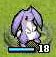# 星之一角

What have you found for these years?

## 2010-07-20

### combinations, list monad and list comprehension

'((A B C) (D E) (F G H))

'((A D F) (A D G) (A D H) (A E F) (A E G) (A E H)
(B D F) (B D G) (B D H) (B E F) (B E G) (B E H)
(C D F) (C D G) (C D H) (C E F) (C E G) (C E H))

for i in xs
for j in ys
for k in zs
for ...

tail recursion, 則很容易再轉成迴圈的方式。

Edward 說他用了一個 lisp 的 macro + function 寫出來了。

`combos0 :: [[a]] -> [[a]]combos0 [] = [[]]combos0 (xs:xss) = ...`

`concatMap (...) (combos0 xss)`

lambda 進去的那邊，會得到其中一個 result list, 這邊寫作 rs:
`concatMap (\rs -> ...) (combos0 xss)`

`x:rs`

`map (...) xs`

`\rs -> map (:rs) xs`

`combos0 (xs:xss) = concatMap (\rs -> map (:rs) xs) (combos0 xss)`

x : rs, 而 x 來自 xs, rs 來自 combos1 xss.
`combos1 (xs:xss) = [ x : rs | x <- xs, rs <- combos1 xss ]`

`[   (x, y) | x <- [1..2], y <- [3..4] ]do{          x <- [1..2]; y <- [3..4];  return (x, y) }`

`do{ x <- xs; rs <- combos2 xss; return (x : rs) }`

`combos3 :: [[a]] -> [[a]]combos3 [] = [[]]combos3 (xs:xss) = xs `monadic_cons` (combos3 xss) where                       monadic_cons = liftM2 (:)`

`xs `monadic_cons` (xs' `monadic_cons` (xs'' `monadic_cons` ...))`

`combos :: [[a]] -> [[a]]combos = foldr (liftM2 (:)) [[]]`

combos.hs

#### 0 retries: